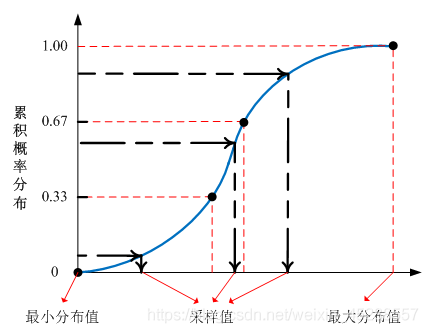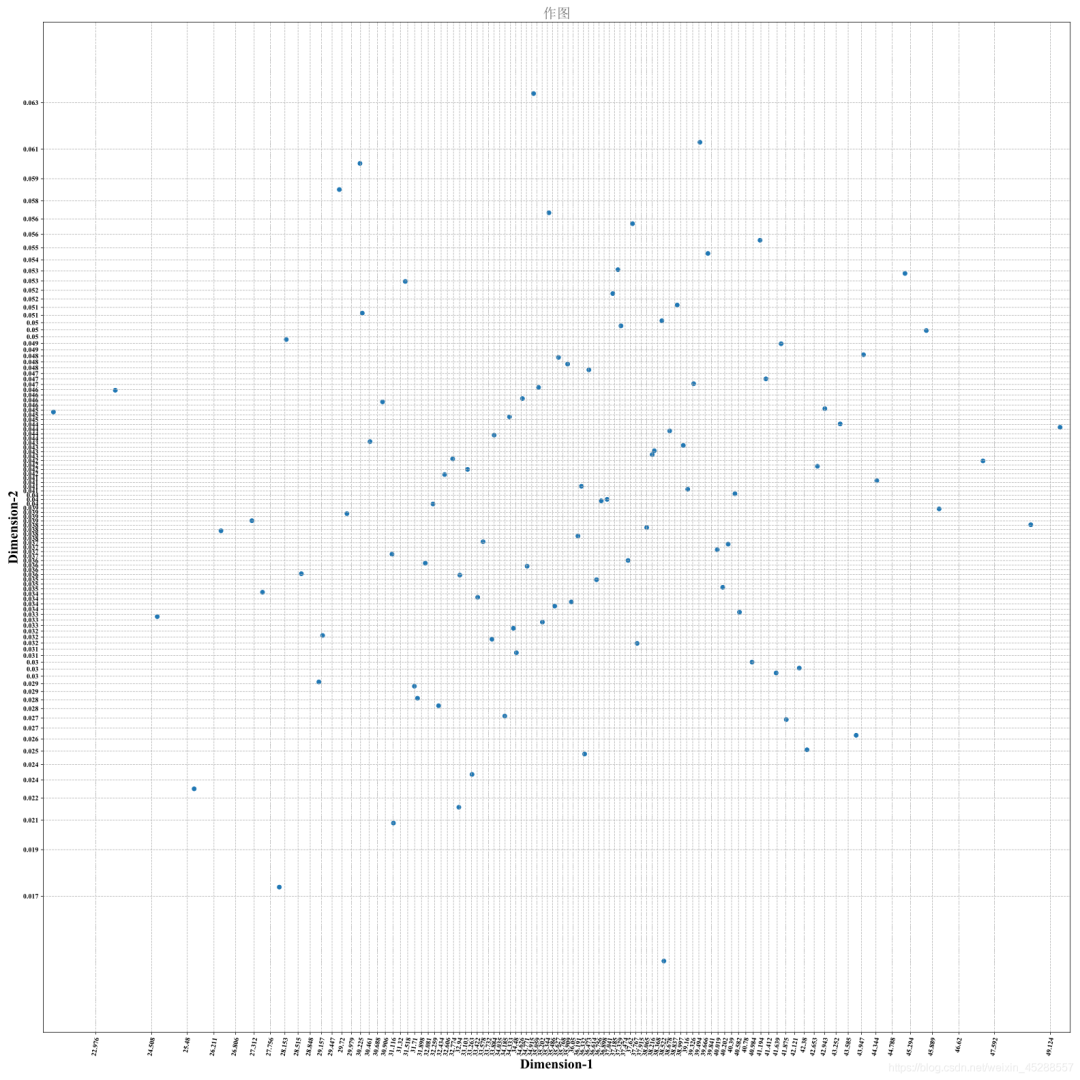# 对应于正态分布的拉丁超立方抽样

2020-09-11 20:23 BalMind团队

0、拉丁超立方抽样的理论基础

## 0.1、概况

• 中值拉丁超立方抽样法

• 拉丁超立方重要抽样法

• 含随机排序法的拉丁超立方抽样

y=f(x)

## 0.3、基本步骤

1. 将每一维向量空间分成N份，根据式上式的反函数求得对应区间，使得每个区间具有相同的概率；

2. 在每一维的每个区间中随机选取一个点作为采样点；

3. 对每一维空间选出的样本点进行随机排序组成各自向量；

4. 将上面采集到的样本向量进行组合就得到一个k×N的样本矩阵。图2-抽样结果实例图像展示

1、导入库和基本准备

#开头的基础设置from __future__ import divisionimport mathimport numpy as npimport matplotlib.pyplot as plfrom numpy.random import RandomState #引入伪随机数器import scipy.stats as st #引入统计函数库，为了引出累计函数的逆函数#获取随机参数数据D = 2 #参数个数N = 100 #拉丁超立方层数，最终生成的数组个数result = np.empty([N, D]) # N行D列的随机数组e = np.empty([N, D]) # N行D列的随机数组---为了记录每个等概率区域边界的随机变量值temp = np.empty([N])      #一维随机数组emp = np.empty([N])       #一维随机数组---为了记录每个等概率区域边界的随机变量值#print(result,e,temp) #检查到这里之前阶段代码的正确性d = 1.0 / N

# 2.1、生成第一个参数的随机数

# 第1个参数# 正态分布的loc=mu and scale=sigmamu= 36.05sigma= 5.62 random_state=111                       #伪随机数器的“种子” rdm = RandomState(random_state)#   j代表一个区域一个区域循环---（等概率区域）for j in range(N):    #设置等概率（=1/N）区域    e1 = st.norm.ppf(j*d,mu,sigma)    e2 = st.norm.ppf((j+1)*d,mu,sigma)    emp[j]=e2    s = rdm.normal(mu, sigma)    # 如果生成的随机数在e1和e2之间即满足了符合要求分布的所在区域的随机数    while s<e1 or s>e2:    #基于伪随机数器的正态分布         s = rdm.normal(mu, sigma)    temp[j] = s#shuffle(x)---现场修改序列，改变自身内容。（类似洗牌，打乱顺序）np.random.shuffle(temp)    for j in range(N):    result[j, 0] = temp[j]    e[j, 0] = emp[j]

2.2、生成第二个参数的随机数

#第2个参数# 正态分布的loc=mu and scale=sigmamu= 0.04sigma= 0.01 random_state=222                       #伪随机数器的“种子” rdm = RandomState(random_state)#=======j代表一个区域一个区域循环---（等概率区域）for j in range(N):    #设置等概率（=1/N）区域    e1 = st.norm.ppf(j*d,mu,sigma)    e2 = st.norm.ppf((j+1)*d,mu,sigma)    emp[j]=e2    s = rdm.normal(mu, sigma)    # 如果生成的随机数在e1和e2之间即满足了符合要求分布的所在区域的随机数    while s<e1 or s>e2:    #基于伪随机数器的正态分布         s = rdm.normal(mu, sigma)    temp[j] = s#shuffle(x)---现场修改序列，改变自身内容。（类似洗牌，打乱顺序）np.random.shuffle(temp)    for j in range(N):    result[j, 1] = temp[j]    e[j, 1] = emp[j]

3、将生成的随机数输出到Excel中

#将数据输出到excel中import xlsxwriterworkbook = xlsxwriter.Workbook('result.xlsx') # 建立文件worksheet1 = workbook.add_worksheet('第1个') # 建立sheet，worksheet2 = workbook.add_worksheet('第2个') # 可以work.add_worksheet('employee')来指定sheet名for i in range(N):    worksheet1.write(0,i,result[i,0])#X = result[:,0]---绘图的X轴    worksheet2.write(0,i,result[i,1])#Y = result[:,1]---绘图的Y轴    workbook.close()

4、将生成的随机数输出到图像中

pl.figure(figsize=(22,22))    # 设置画布大小pl.grid(linestyle='--')       #设置网格线---这里是虚线#==========字体的引入==========import matplotlib.font_manager as fmmyfont = fm.FontProperties(fname=r"C:\\WINDOWS\\Fonts\\simsun.ttc")  #将所给字体文件转化为此处可以使用的格式，可使用自己电脑自带的其他字体文件，你可以使用自己电脑C:\Windows\Fonts下的字体#===绘制散点图===X = result[:,0]Y = result[:,1]pl.scatter(X,Y)#===图的标题---参数（图的标题，字体，字号， 颜色， 位置），注：颜色取值在0到1之间===pl.title('作图',fontproperties=myfont, fontsize=20, color=(0.4,0.4,0.4), loc='center') #===设置轴坐标刻度值的大小以及刻度值的字体===#***这个地方会报警告——无视（注意版本太新可能未必有这个命令）***ax=pl.subplot(111) #注意:一般都在ax中设置,不在plot中设置，这个是用来设置多个子图的位置,labels = ax.get_xticklabels() + ax.get_yticklabels()[label.set_fontname('Times New Roman') for label in labels]#===设置轴坐标的名称以及对应字体格式===font2 = {'family': 'Times New Roman',         'weight': 'normal',         'size': 20,         }pl.xlabel('Dimension-1', font2)pl.ylabel('Dimension-2', font2)#===设置轴坐标的其他相关设置===para1=e[:-1,0]   #因为正态分布的随机变量由负无穷到正无穷，而坐标值需要是个具体的值，而记录边界值的数组e 不是，包含"正无穷"点，故需要舍掉才容易表示到图上，para2=e[:-1,1]   # 数组e 的第一个数就是从第一个等概率区域的右边界点开始记录的，故相当于已经舍掉“负无穷”点                 #这里再舍掉“正无穷”点-即最后一个数据就是绘图轴坐标数据#print(para)biao_q1=np.around(para1, decimals=3) #numpy.around() 函数---要舍入的小数位数（默认值：0）,如果小数为负数，则指定小数点左边的位数。1. 可以传递给Decimal整型或者字符串参数，但不能是浮点数据，因为浮点数据本身就不准确。2. Decimal还可以用来限定数据的总位数。biao_q2=np.around(para2, decimals=3)pl.tick_params(axis='x', labelsize=10)    # 设置x轴标签大小pl.xticks(para1,biao_q1)pl.xticks(rotation=80)    # 设置x轴标签旋转角度pl.tick_params(axis='y', labelsize=10)    # 设置y轴标签大小pl.yticks(para2,biao_q2)pl.tight_layout()#自动调整标签使之不重叠，---“整个布局自适应”#绘图并保存图片，且注意savefig和show的顺序一定不能变pl.margins(0.01,0.03)  #去除空白fig = pl.gcf()pl.show()fig.savefig('LHS.svg', dpi=300) #dpi是设置清晰度的，大于300就比较清晰